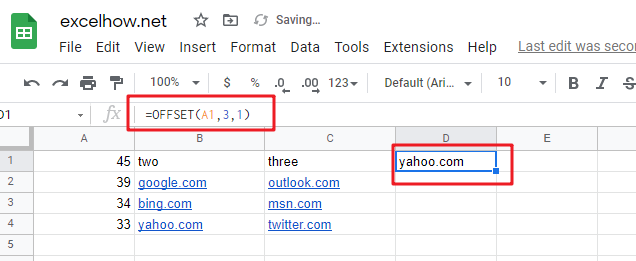## Calculate Average Of Last 5 Or N Values In Columns in Google Sheets

Suppose you come across a task where you need to calculate the average of the last 2 or 3 numeric values in google sheets, then what would you do?

If you are new to google sheets, then your first attempt might be doing this task manually, which is an acceptable way but only when the values of which you want to calculate the average is limited to 2 or 3 but when it comes to calculating the last 5 or N values in the columns then it becomes nearly impossible to do this cumbersome task on time!

Don’t worry about it because after reading this article, you will know the easiest way to calculate the average of the last 5 or N values from the columns within seconds.

So, let’s dive into the article.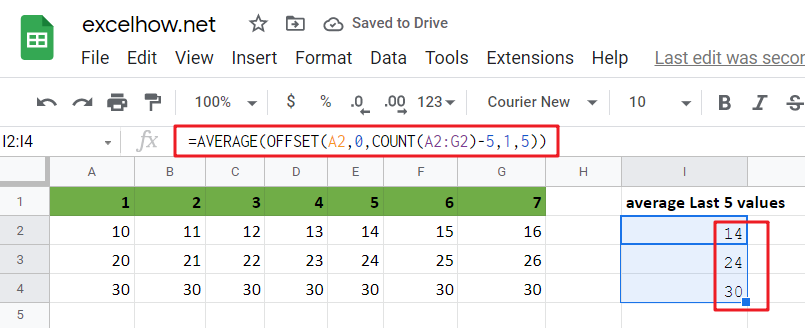## General Formula in google sheets

To get the average of the last N values in google sheets, use the formula below.

`=AVERAGE(OFFSET(first cell,0,COUNT(range_values)-N,1,N))`

### Explanations For Syntax:

• AVERAGE: In google sheets, the AVERAGE Function may be used to calculate the arithmetic mean of a set of integers.
• OFFSET: This Function outputs a reference to a range made up of pieces such as a beginning point, a row, and column offset, and a final height and width in rows and columns. Learn more about the OFFSET Function.
• COUNT: The COUNT Function returns the result as a number and counts the number of cells that contain numbers.
• First Cell: It indicates the first cell in the provided input range.
• The comma sign (,): is a separator that separates a list of values.
• Parenthesis (): The main Function of this symbol is to group the elements.
• Minus Operator (-): This symbol subtracts 2 values.

### Explanation

To average the latest 5 data values in a range of columns in google sheets, use the AVERAGE Function conjunction with the COUNT and OFFSET functions. The formula in I2 in the example is as follows:

`=AVERAGE(OFFSET(A2,0,COUNT(A2:G2)-5,1,5))`The OFFSET function may create dynamic ranges from a beginning cell and specify rows, columns, height, and width.

The rows and columns parameters act as “offsets” from the beginning reference. The height and width parameters, all optional, decide how many rows and columns are included in the final range. We want the OFFSET function to return a range that starts at the last item and grows “backward,” therefore we provide the following arguments:

A2 is the initial reference — it is the cell directly to the right of the formula and the first cell in the range of values we are dealing with.

Rows – We use 0 for the rows option because we want to stay in the same row.

Columns – We use the COUNT function to count all values in the range for the columns input, then deduct 5. This moves the start of the range of 5 columns to the left.

Height – we use 1 since we want a 1-row range as the end result.

Width – we pick 5 since we want a final range with 5 columns.

For the formula in I2, OFFSET yields a final range of C2:G2. This is sent to the AVERAGE Function, which returns the average of the five values in the range.

To understand better, consider the following example, which follows a step-by-step procedure:

• Consider the following example to calculate the average of the last 5 values.
• This illustration will provide input data from Column A to Column G.
• Next, enter the supplied formula in the formula bar section.
• Finally, we shall obtain the result in the selected cell I2.

## Less Than 5 Values Average in google sheets

If you are want to compute the average of the last 2, 3, or 4 data in google sheets, you don’t need to do it manually. Utilizing the formula discussed above will result in a circular reference mistake when computing the average of the 2, 3, or 4 values in the columns; the range will extend back into the cell that contains the formula. To avoid this mistake and complete your task, modify the formula as follows:

`=AVERAGE(OFFSET(first,0,COUNT(rng)-MIN(N,COUNT(rng)),1,MIN(N,COUNT(rng))))`

So If you want to average last N values in columns that less than 5 values in google sheets, you can use the following formula:

`=AVERAGE(OFFSET(A2,0,COUNT(A2:C2)-MIN(5,COUNT(A2:C2)),1,MIN(5,COUNT(A2:C2))))`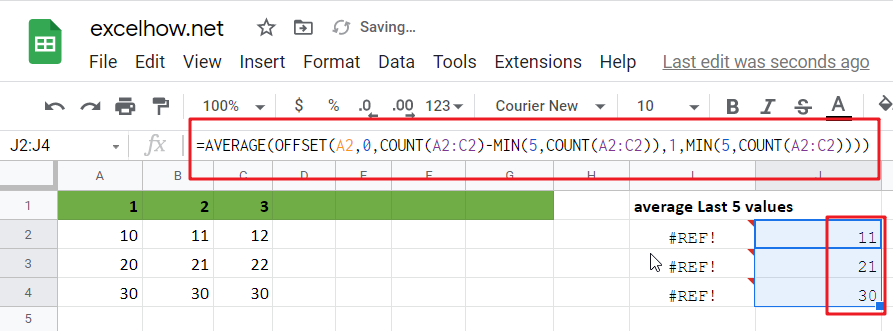In this case, we utilize the MIN function to “catch” cases where there are fewer than 5 values and the real count when there are.

### Related Functions

The Google Sheets AVERAGE function returns the average of the numbers that you provided.The syntax of the AVERAGE function is as below:=AVERAGE (number1,[number2],…)….
The Google Sheets  COUNT function counts the number of cells that contain numbers, and counts numbers within the list of arguments. It returns a numeric value that indicate the number of cells that contain numbers in a range…
The Google Sheets OFFSET function returns a range reference shifted a specified number of rows and columns from a starting cell reference.The syntax of the OFFSET function is as below:= OFFSET(cell_reference, offset_rows, offset_columns, [height], [width])…
The Google Sheets MIN function returns the smallest numeric value from the numbers that you provided. Or returns the smallest value in the array.The MIN function is a build-in function in Google Sheets and it is categorized as a Statistical Function.The syntax of the MIN function is as below:= MIN(num1,[num2,…numn])….

## Average Last N Values in Google Sheets

Average is a valuable function in google sheets that allows you to rapidly compute the average of the past N values in a column. However, you may need to insert new numbers beneath your original information from time to time, and you just want the average result to be updated automatically when new data is entered. This means you would like the average to always represent the latest N values in your data list, regardless of whether or not you add new data points.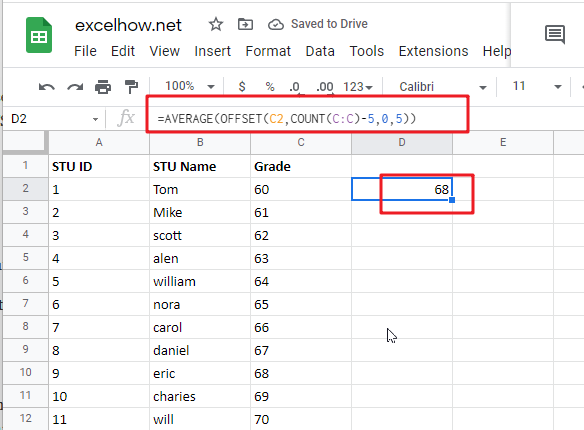The Generic formula is as follow:

`=AVERAGE(OFFSET(C2,COUNT(C:C)-N_Values,0,N_Values))`

## Summary

The AVERAGE function, in conjunction with the COUNT and OFFSET functions, may be used to calculate an average of the latest 5 data points. You may use this strategy to average the most recent N data points, such as the most recent 3 days, the most recent 6 measures, and so on. The following is the formula in F6 in the case shown:

`=AVERAGE(OFFSET(C2,COUNT(C:C)-5,0,5))`## Let’s See How This Formula Works

This function may be used to create dynamic rectangular ranges using a beginning reference and the values of the rows, columns, height, and width parameters. The rows and columns parameters are treated as “offsets” from the initial reference in the same manner. The number of rows and columns in the final range is determined by the height and width parameters (both of which are optional). For the sake of this example, OFFSET is set as follows:

• reference = C2
• rows = COUNT(C:C)
• cols = 0
• height = -5
• width = (not provided)

The beginning reference is supplied as C2, which is the cell immediately above the actual data in the table. Because we want OFFSET to provide a range of values starting with the last item in column C. We use the COUNT function to count all of the values in column C to get the needed row offset for OFFSET. COUNT solely counts numeric numbers, which means that the heading in row 1 is discarded automatically.

OFFSET resolves to the following when there are 10 numerical values in column C:

`=OFFSET(C2,COUNT(C:C)-5,0,5)`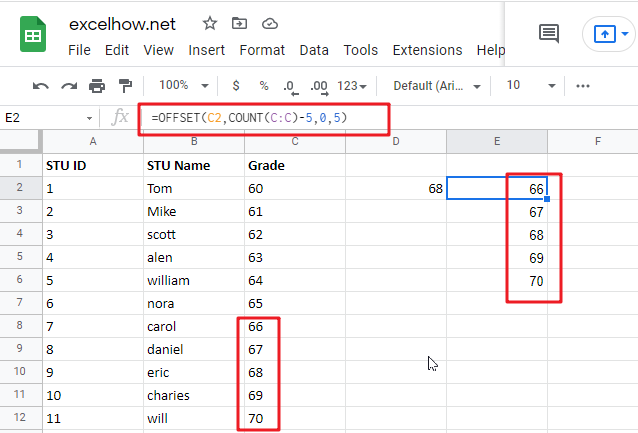This combination of parameters begins at C2, offsets 11 rows to C12, and then uses -5 to expand the rectangular range up “backwards” 5 rows to form the range C8:C12, which is the range C2:C12.

At the end of the process, the OFFSET function passes the range C8:C12 to the AVERAGE function, which computes the average values in the range.

## With a dynamic range

For the sake of simplicity, the example in this section utilises a complete column reference for the data that is being averaged – when new information is added to column C. The formula will continue to function correctly since reference C includes the whole column C. However, you may also utilise a dynamic range in the following manner:

` =AVERAGE(OFFSET(C2,COUNT(DATA_Range)-5,0,5))`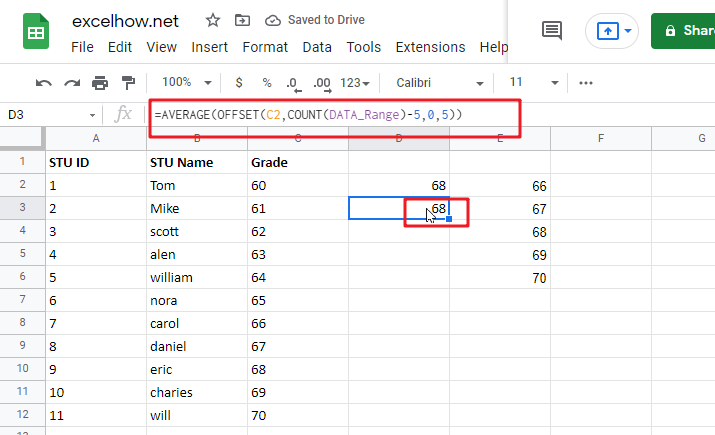Where ” `DATA_Range` ” refers to a dynamic named range or a reference to a column in an google sheets Table, respectively. google sheets Tables and dynamic named ranges can dynamically expand as new data is entered, which is crucial for using this method. Take note that the reference to OFFSET is still in the row before the data appears.

### Related Functions

The Google Sheets AVERAGE function returns the average of the numbers that you provided.The syntax of the AVERAGE function is as below:=AVERAGE (number1,[number2],…)….
The Google Sheets  COUNT function counts the number of cells that contain numbers, and counts numbers within the list of arguments. It returns a numeric value that indicate the number of cells that contain numbers in a range…
The Google Sheets OFFSET function returns a range reference shifted a specified number of rows and columns from a starting cell reference.The syntax of the OFFSET function is as below:= OFFSET(cell_reference, offset_rows, offset_columns, [height], [width])…

This post will guide you how to use Google Sheets OFFSET function with syntax and examples. Most of google spreadsheet users are very confusing and don’t know how this function works in google sheets. After leaning this function, you should know that this function is very useful and you can read the below guide to understand how and why this function is very useful.

## Description

The Google Sheets OFFSET function returns a range reference shifted a specified number of rows and columns from a starting cell reference.

The OFFSET function can be used shift a range of a certain number of rows or columns in google sheets. The purpose of this function is to create a reference offset from a given starting cell reference and It’s returned value is a cell reference.

The OFFSET function is a build-in function in Google Sheets and it is categorized as a LOOKUP function.

## Syntax

The syntax of the OFFSET function is as below:

= OFFSET(cell_reference, offset_rows, offset_columns, [height], [width])

Where the OFFSET function arguments are:

• cell_reference -This is a required argument. The starting point from which to count the offset rows and columns.
• offset_rows – This is a required argument. The number of rows to offset to shift by.
• offset_columns – This is a required argument. The number of columns to offset to shift by.
• height – This is an optional argument. The height of the range to return starting at the offset target.
• width – This is an optional argument. The width of the range to return starting at the offset target.

Note:

• If offset_rows is a negative value, it is possible for the offset target to be outside of the spreadsheet. If this occurs, OFFSET function will return the #REF! error.
• If offset_columns is a negative value, it is possible for the offset target to be outside of the spreadsheet. If this occurs, OFFSET function will return the #REF! error.
• OFFSET function only returns a cell reference

## Google Sheets OFFSET Function Examples

The below examples will show you how to use google sheets OFFSET Function to shift a range of a certain number of rows or columns.

#1 =OFFSET(A1,3,1)

This OFFSET Formula will return the forth value in the second column, see the below screenshot: